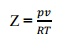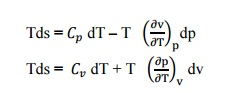Home | | Engineering Thermodynamics | Important Questions and Answers: Ideal And Real Gases, Thermodynamic Relations

# Important Questions and Answers: Ideal And Real Gases, Thermodynamic Relations

Mechanical - Engineering Thermodynamics - Ideal And Real Gases, Thermodynamic Relations

IDEAL AND REAL GASES, THERMODYNAMIC RELATIONS

Avogadro’s law states, “Equal volumes of d and pressure, contain equal number of molecu

2.  State   Dalton’s   law partial  pressure?

Dalton’s law of partial pressure state,” T sum of the partial pressures exerted by individual gases if each one of them occupied

separately in the total volume of the mixture at mixture temperature”.

3.     How does the Vander waals equation differ from the ideal gas equation of state?

·        Intermolecular attractive study is made.

·        Shape factor is considered.

These assumptions are not made in ideal gas equation of state.

4.     What is meant by virtual expansion?[A

Viral or Virtual expansions are only applicable to gases of low and medium densities. The equation state of a substance is given by,The coefficient ofevirala(T),coefficients. Theb(T),c(T)viralcoefficientwill…. ar vanish when the pressure becomes zero. Finally, the equation of state reduces to the ideal-gas

equation.

5.  Distinguish between ideal and real gas?

An ideal gas is one which strictly follows the gas laws under all conditions of temperature and pressure.

In actual practice, there is no real gas which strictly follows the gas laws over the entire range of temperature and pressure. However, hydrogen, oxygen, nitrogen and air behave as an ideal gas under certain temperature and pressure limits.

6.     What are Maxwell relations?

There are four Maxwell relations,These are the Maxwell relations.

7.  Define Joule–Thomson co–efficient?

Joule –Thomson co –efficient is defined as the change in temperature with change in pressure, keeping the enthalpy remains constant. It is denoted by,7.     Define co-efficient of volume expansion and isothermal compressibility?

Co-efficient of volume expansions is defined as the change in volume with change in

temperature per unit volume keeping the pressure constant. It is denoted by,Isothermal compressibility is defined as the change in volume with change in pressure per unit volume by keeping the temperature constant. It is denoted by,8.     What is compressibility factor?

We know that, the perfect gas equation is pv=RT. But for real gas, a correction factor hasto be introduced in the perfect gas equation to take into account the deviation of real gas from the perfect gas equation. This factor is known as compressibility factor (Z) and is defined by,10.                        What is compressibility factor? What does it signify? What is its value for an ideal das at critical point?

We know that, the perfect gas equation is pv=RT. But for real gas, a correction factor has to be introduced in the perfect gas equation to take into account the deviation of real gas from the perfect gas equation. This factor is known as compressibility factor(Z) and is defined by,

·        Intermolecular attractive study is made.

·        Shape factor is considered.

At      critical   point,ation   the   Vander   waal’s   equ10.                        Draw the generalized compressibility chart?12.                        What is Joule Thomson coefficient? Why is it zero for an ideal gas?

Joule –Thomson co –efficient is defined as the change in temperature with change in pressure, keeping the enthalpy remains constant. It is denoted by,

We know that the equation of state as pV =RT

Differentiating the above equation of state with respect to T by keeping pressure, p constant.Therefore, µ = 0.

It implies that the Joule-Thomson coefficient is zero for ideal gas.

13. What is Clasius Clapeyron Equation?

Clapeyron equation involves relationship between the saturation temperature, saturation pressure, the enthalpy of evaporation and the specific volume of the two phases involved.14.                        State Tds Equations?

Tds Equations are,15. State Helmholtz function?

Helmholtz function is property of a system and is given by subtracting the product of absolute temperature (T) and entropy (s) from the internal energy u.

i.e. Helmholtz function = u –Ts

16. Explain the Amagat’slaw of partial volume.

The total volume of mixture of gases is equal to the sum of the partial volumes of the components. The partial volume of a component will be the volume it will occupy at the total pressure at that temperature.

17. Explain   Boyle’s   law.

It states that for given mass of gas at constant temperature, the pressure is inversely proportional to its volume.

18. Explain   Charle’s   law.

It states that for a given mass of gas at constant pressure, its volume varies directly as its absolute temperature.

19. Explain   Gay   Lussac’s   law.

It states that for a given mass of gas at constant volume, its pressure is directly proportional to its absolute temperature

Study Material, Lecturing Notes, Assignment, Reference, Wiki description explanation, brief detail
Mechanical : Engineering Thermodynamics : Ideal And Real Gases, Thermodynamic Relations : Important Questions and Answers: Ideal And Real Gases, Thermodynamic Relations |